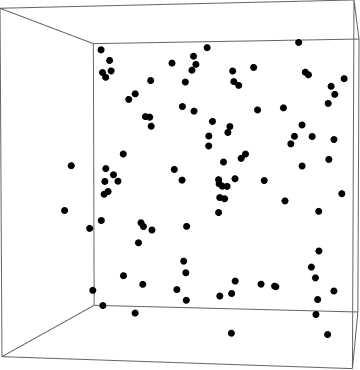# Fluctuations in the ideal gas

The well-known equation of state for an ideal gas, $PV = Nk_BT,$ is only true on average. In reality, the pressure fluctuates from moment to moment as more or fewer gas particles hit the wall in a given instant.Suppose we measure the pressure on the walls of the container at $m$ different times $\mathcal{P} = \{P_{t_1}, P_{t_2},\ldots, P_{t_m}\}.$ We can measure the magnitude of the pressure fluctuations by finding the standard deviation $\sigma_P = \sqrt{\langle P^2\rangle - \langle P\rangle^2}.$

How will the size of the fluctuations shrink relative to the mean as we increase the number of gas particles?

In other words, if $N$ denotes the number of particles, what is $\dfrac{\sigma_P}{\langle P\rangle}$ proportional to?

×